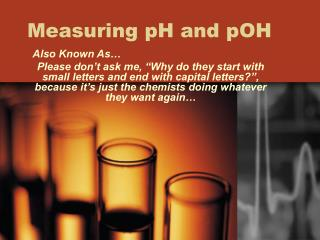DownloadDownload PresentationMeasuring pH and pOH

# Measuring pH and pOH

Download Presentation## Measuring pH and pOH

- - - - - - - - - - - - - - - - - - - - - - - - - - - E N D - - - - - - - - - - - - - - - - - - - - - - - - - - -
##### Presentation Transcript

1. Measuring pH and pOH Also Known As… Please don’t ask me, “Why do they start with small letters and end with capital letters?”, because it’s just the chemists doing whatever they want again…

2. What is “pH”? • The term “pH” refers to the measurement of the strength of an acid or base. • “pH” comes to us from “portenz of hydrogen” – portenz means strength and the hydrogen’s chemical symbol is H. (p + H = pH) • pH is measured using the pH scale which runs numerically from 0 to 14. • 0 – 7 is the region of acids (acidic solutions) • 7 is perfectly neutral (as pure water would be) • 7-14 is the region of bases (alkaline solutions)

3. The pH Scale • The pH scale ranges from 0-14. A pH of 7 is perfectly neutral like pure water. • As you go away from seven and count down toward zero – you are getting more and more acidic. • As you count up from seven toward fourteen – you are getting more and more basic. • The pH scale is based on a logarithm to the base 10 which means that each step along the pH scale represents a change in the strength of the acid or base by 10x. • So, for acids, an acid with a pH of 3 is ten times stronger than an acid of pH 4. (101) • An acid with a pH of 2 is one thousand times stronger than an acid of pH 5. (103 = 10 x 10 x 10) • Bases operate by the same rule of measure.

4. pIC of the pH Scale

5. Calculating pH (Part I) • pH measures the amount of hydrogen ions released by the acid when placed in a solution. (Recall the link between dissociation and acid/base strength from previous lecture.) • When an acid is placed in a solution, it releases the hydrogen (H+) ions and these ions attach themselves to the water molecules (H2O) to make the hydronium ion (H3O+). The more hydronium ions that are made – the more H+ ions that must have been released – the stronger the acid. H+ + H2O  H3O+ • This means that to measure the pH of an acid, we are actually measuring the number of hydronium ions rather than the number of hydrogen ions because the hydrogen ions quickly form hydroniums.

6. Calculating pH (Part II) • The formula for calculating ph is: pH = – log10[H3O+] • The square brackets around the hydronium means that you are using the concentration of hydronium ions in the solution being measured. • The next step is figuring out how to use your calculator to solve problems using this logarithmic relationship – each calculator will have its own order of button-pushing to follow so now you must learn to use your calculator…No really…I’m serious.

7. Down Wit Da pOH pOH’s • The pH measures the strength of an acid but it does not do the same for a base. • A base is a hydrogen ion acceptor so when you put it in water – it doesn’t form the hydronium ion – it forms the hydroxide ion (OH–) because it takes an H+ from the water. H2O – H+ OH– • This means that the accurate way to measure the strength of a base is to measure the number of hydroxide ions because the base molecules steal H+ ions from the water and quickly turn the water molecules into hydroxide ions (OH–).

8. Calculating the pOH • The formula for pOH is: pOH = – log10[OH–] • Again, the squared brackets tells you the value within them is the concentration of the hydroxide ions in the solution being measured. • And again, the calculator should be the toughest part of figuring out how to solve these problems.

9. Connecting pH to pOH • So…pH measures the strength of an acid and pOH measures a base. Why then do we use pH to measure a base on the pH scale? • We convert pOH into pH because of the relationship between the two values – it is shown below: pH + pOH = 14 • This means that if we are given the concentration of hydronium ions in an acid – we find the pH and use that value to describe its strength. • If we are given the concentration of hydroxide ions in a base – we find the pOH and use the relationship above to convert the pOH into a pH value. • So easy…well…except for that calculator stuff…

10. THE END